Courses

RD Sharma Solutions - Ex - 4.3 & Ex - 4.4, Rational Numbers, Class 7, Math Notes | Study RD Sharma Solutions for Class 7 Mathematics - Class 7

Class 7: RD Sharma Solutions - Ex - 4.3 & Ex - 4.4, Rational Numbers, Class 7, Math Notes | Study RD Sharma Solutions for Class 7 Mathematics - Class 7

The document RD Sharma Solutions - Ex - 4.3 & Ex - 4.4, Rational Numbers, Class 7, Math Notes | Study RD Sharma Solutions for Class 7 Mathematics - Class 7 is a part of the Class 7 Course RD Sharma Solutions for Class 7 Mathematics.
All you need of Class 7 at this link: Class 7

Exercise 4.3

QUESTION 1:

Determine whether the following rational numbers are in the lowest form or not:

(i) 65/84
(ii) −15/32
(iii) 24/128
(iv) −56/−32

(i) We observe that 65 and 84 have no common factor i..e., their HCF is 1.

Thus, 65/84 is in its lowest form.

(ii) We observe that −-15 and 32 have no common factor i..e., their HCF is 1.

Thus, −15/32 is  in  its lowest form.

(iii) HCF of 24 and 128 is not 1.

Thus, given rational number is not in its simplest form.

(iv) HCF of 56 and 32 is 8.

Thus, given rational number is not in its simplest form.

QUESTION 2:

Express each of the following rational numbers to the lowest form:

(i) 4/22
(ii) −36/180
(iii) 132/−428
(iv) −32/−56

Lowest form of:
(i) 4/22 is:
4 =2×2
22 = 2×11
HCF of 4 and 22 is 2.
Dividing the fraction by 2 , we get  2/11.

(ii)  −36/180 is:
36 = 3×3×2×2
180 = 5×3×3×2×2
HCF of  36 and 180 is 36.
Dividing the fraction by 36, we get −1/5

(iii) 132/−428 is:
132 = 2×3×2×11
428 = 2×2×107
HCF of 132 and 428 is 4.
Dividing the fraction by 4 , we get   33/−107

(iv) −32/−56 is:
32 =2×2×2×2×2
56 = 2×2×2×7
HCF of 32 and 56 is 8.
Dividing the fraction by 8, we get 4/7

QUESTION 3:

Fill in the blanks: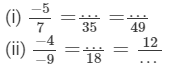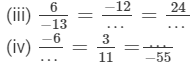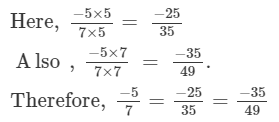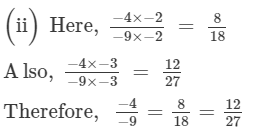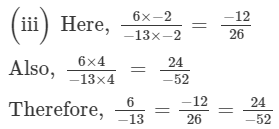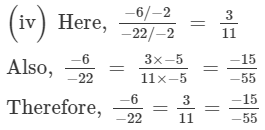Exercise 4.4

QUESTION 1:

Write each of the following rational numbers in the standard form: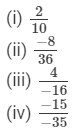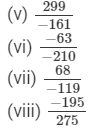(i) The denominator is positive and HCF of 2 and 10 is 2.

Dividing the numerator and denominator by 2, we get: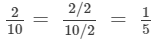(ii) The denominator is positive and HCF of 8 and 36 is 4.

Dividing the numerator and denominator by 4, we get: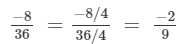(iii)
The denominator is negative.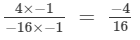HCF of 4 and 16 is 4.
Dividing the numerator and denominator by 4, we get: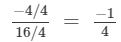(iv) The denominator is negative.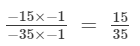HCF of 15 and 35 is 5.
Dividing the numerator and denominator by 5, we get: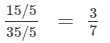(v) The denominator is negative.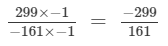HCF of 299 and 161 is 23.

Dividing the numerator and denominator by 23, we get: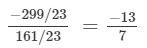(vi) The denominator is negative.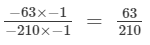HCF of 63 and 210 is 21.

Dividing the numerator and denominator by 21, we get: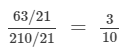(vii) The denominator is negative.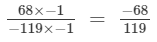HCF of 68 and 119 is 17.

Dividing the numerator and denominator by 17, we get: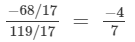(viii) The denominator is positive and HCF of 195 and 275 is 5.

Dividing divide the numerator and denominator by 5, we get: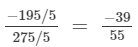The document RD Sharma Solutions - Ex - 4.3 & Ex - 4.4, Rational Numbers, Class 7, Math Notes | Study RD Sharma Solutions for Class 7 Mathematics - Class 7 is a part of the Class 7 Course RD Sharma Solutions for Class 7 Mathematics.
All you need of Class 7 at this link: Class 7Use Code STAYHOME200 and get INR 200 additional OFF Use Coupon Code

Top Courses for Class 7RD Sharma Solutions for Class 7 Mathematics

97 docs

Top Courses for Class 7Track your progress, build streaks, highlight & save important lessons and more!

,

,

,

,

,

,

,

,

,

,

,

,

,

,

,

,

,

,

,

,

,

,

,

,

,

,

,

,

,

,

;